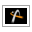AstroGrav Help /Data Elements /Rectangular ElementsVersion 5.0.1

#Rectangular Elements

An object's rectangular elements are the position and velocity components relative to the object's parent.

• The Orbital Position is the vector that describes the position relative to the object's parent.

• The Orbital Velocity is the vector that describes the velocity relative to the object's parent.

The rectangular elements are as follows.

• Orbital Position X is the x-coordinate of the orbital position, and has the symbol rx.

The x-coordinate of the orbital position may be any number.

• Orbital Position Y is the y-coordinate of the orbital position, and has the symbol ry.

The y-coordinate of the orbital position may be any number.

• Orbital Position Z is the z-coordinate of the orbital position, and has the symbol rz.

The z-coordinate of the orbital position may be any number.

• Orbital Velocity X is the x-coordinate of the orbital velocity, and has the symbol vx.

The x-coordinate of the orbital velocity may be any number.

• Orbital Velocity Y is the y-coordinate of the orbital velocity, and has the symbol vy.

The y-coordinate of the orbital velocity may be any number.

• Orbital Velocity Z is the z-coordinate of the orbital velocity, and has the symbol vz.

The z-coordinate of the orbital velocity may be any number.